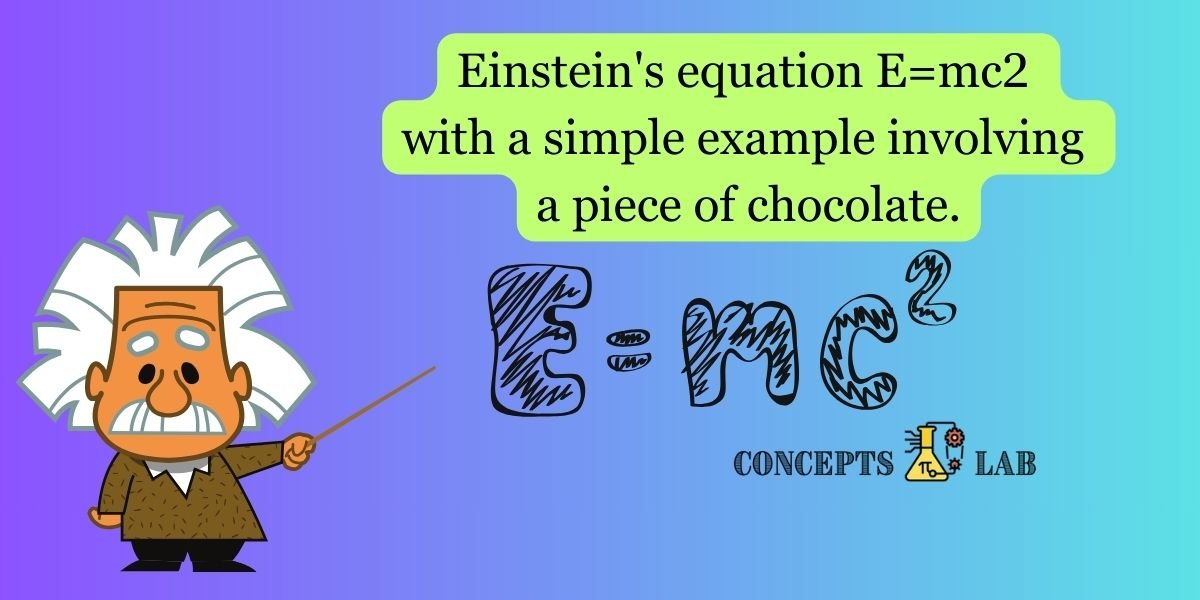Categories:

Einstein’s Energy Chocolate:

1. Energy (E):
• Think of energy (E) as the delicious taste and sweetness of a piece of chocolate. The more energy, the tastier it is!
2. Mass (m):
• The mass (m) is the actual amount of chocolate. If you have a big piece, it has more mass. If it’s a small piece, it has less mass.
3. Speed of Light (c):
• The speed of light (c) is like a magical number that’s really, really big – about 300,000 kilometers per second. In our example, cc represents the speed at which light travels.

Putting it Together: Now, let’s use Einstein’s equation to understand how much energy is hidden in the mass of the chocolate.

``````E=mc2
``````

Example: Chocolate Magic: Imagine you have a piece of chocolate. Let’s say the mass (mm) of the chocolate is 100100 grams (just for illustration purposes).

``````E=(100grams)×(300,000km/s)2

``````

The speed of light squared is an enormous number, and when you multiply it by the mass, you get a large amount of hidden energy.

Real-Life Magic: This hidden energy is in the form of mass-energy equivalence, as described by Einstein. Even when the chocolate is just sitting there, not moving or melting, there’s a bit of hidden magic inside it.

Eating the Chocolate: Now, when you take a bite of the chocolate, you’re releasing some of that hidden energy. It’s like turning a tiny bit of the chocolate’s mass into the delightful taste and energy that you experience.

So, children, every time you enjoy a piece of chocolate, you can remember Einstein’s equation at work, revealing the hidden magic within the mass of the chocolate. It’s a tasty way to understand a bit of the incredible science that surrounds us! 🍫💫